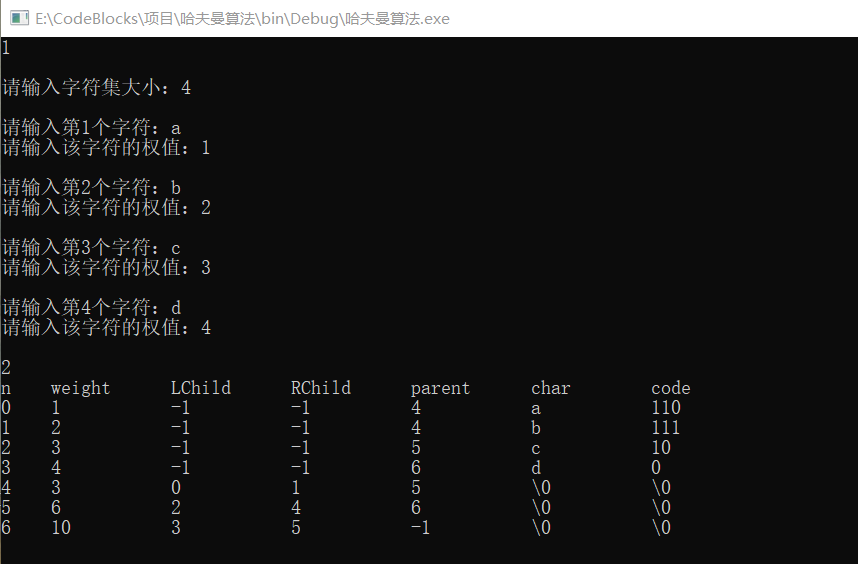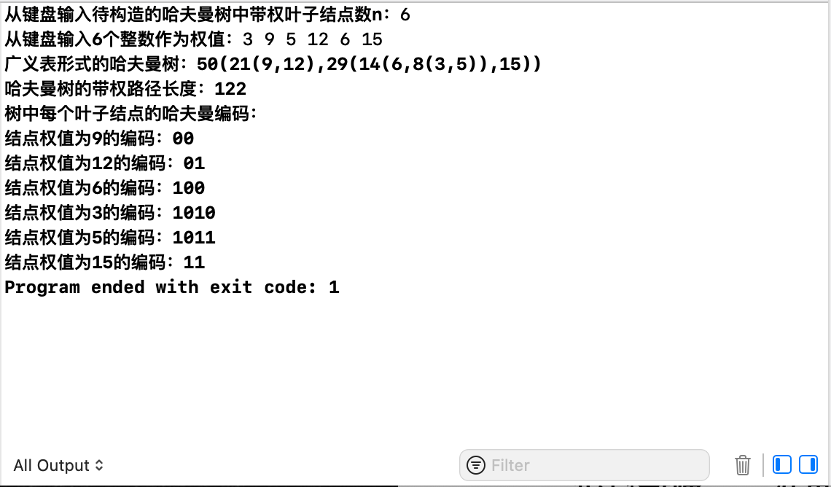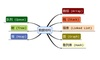• 北邮数据结构实验哈夫曼树，含有报告以及源代码程序
• 数据结构哈夫曼树实验报告

千次阅读 2021-12-05 23:11:53
进一步理解哈夫曼树的逻辑结构和存储结构，进一步提高使用理论知识指导解决实际问题的能力 要求：(1).假设文档内容从键盘输入； (2).设计哈夫曼算法的存储结构； (3).设计哈夫曼编码和解码算法； (4).分析使劲按...
1. 实验目的及要求

目的：熟练掌握二叉树应用（Huffman编码）的基本算法实现；进一步理解哈夫曼树的逻辑结构和存储结构，进一步提高使用理论知识指导解决实际问题的能力

要求：(1).假设文档内容从键盘输入；

(2).设计哈夫曼算法的存储结构；

(3).设计哈夫曼编码和解码算法；

(4).分析使劲按复杂度和空间复杂度。

1. 实验步骤

1.实验问题分析

程序是通过利用二叉树结构实现哈夫曼编码和译码，并且程序需具有以下要求：

（1）初始化：能够让用户输入字符和相应字符的频度来初始化，并建立哈夫曼树。

（2）建立编码表：利用已经建好的哈夫曼树进行编码，并将每个字符的编码进行输出，打印哈夫曼编码表。

（3）译码：利用已经建好的哈夫曼树对编码后的字符串进行译码，并输出译码结果。

运用哈夫曼算法的相关知识解决问题。以用户输入的字符作为需要编码的字符集合，每个字符对应的频度则作为该字符的权值，构造一棵哈夫曼编码树，规定哈弗曼编码树的左分支代表0，右分支代表1，则从根结点到每个叶子结点所经过的路径组成的0和1的序列为需要加密字符的编码。

2.数据的存储结构设计：

（1）采用静态的三叉链表存放。

算法思想：头指针数组存储哈夫曼编码串，字符型指针用来存放临时的编码串；从叶子节点开始向上倒退，若其为它双亲节点的左孩子则编码标0，否则标1；直到根节点为止，最后把临时存储编码复制到对应的指针数组所指向的内存中。重复，直到所有的叶子节点都被编码完。

1. 设计一个结构体Element保存哈夫曼树中各结点的信息；
1. 实验内容

伪代码：

1.用户输入需要编译的字符和该字符的权值;

2.构造哈夫曼算法。设计一个数组H保存哈夫曼树中各结点的信息;

3.数组H初始化，将所有结点的孩子域和双亲域的值初始化为-1;

4.数组H的前n个元素的权值给定值;

5.调用select函数选择权值最小的根结点进行合并，其下标分别为i1，i2;

6.将二叉树i1，i2合并为一棵新的二叉树;

7.共进行n-1次合并，直到剩下一棵二叉树，这棵二叉树就是哈夫曼树;

时间复杂度：

1.Select函数，时间复杂度O(n);

2.Reverse函数，时间复杂度O(n);

3.HuffmanTree函数，构造哈夫曼树，时间复杂度O(n!);

4.CreateCodeTable函数，构造和输出哈夫曼编码表，时间复杂度O(n);

5.Decode函数，解码，时间复杂度O(n)。

代码的实现如下：

#include<iostream>

#include<string>

#include<iomanip>

using namespace std;

struct Element

{

char ch;

int weight;

int lchild, rchild, parent;

};

struct HCode

{

char  data;

char  code;

};

int Select(Element H[], int i)       //选择两个最小的

{

int min = 11000;

int min1;

for (int k = 0; k<i; k++)

{

if (H[k].weight<min && H[k].parent == -1)

{

min = H[k].weight;

min1 = k;

}

}

H[min1].parent = 1;

return min1;

}

void Reverse(char c[])    //将字符串倒置

{

int n = 0;

char temp;

while (c[n + 1] != '\0')

{

n++;

}

for (int i = 0; i <= n / 2; i++)

{

temp = c[i];

c[i] = c[n - i];

c[n - i] = temp;

}

}

void HuffmanTree(Element H[], int w[], int n)     //构造哈夫曼树

{

int i1 = 0, i2 = 0;

for (int i = 0; i<2 * n - 1; i++)

{

H[i].lchild = -1;

H[i].parent = -1;

H[i].rchild = -1;

}

for (int l = 0; l<n; l++)

{

H[l].weight = w[l];

}

for (int k = n; k<2 * n - 1; k++)

{

int i1 = Select(H, k);

int i2 = Select(H, k);

if (i1>i2)

{

int temp;

temp = i1;

i1 = i2;

i2 = temp;

}

H[i1].parent = k;

H[i2].parent = k;

H[k].weight = H[i1].weight + H[i2].weight;

H[k].lchild = i1;

H[k].rchild = i2;

}

}

void CreateCodeTable(Element H[], HCode HC[], int n)      //输出哈弗曼编码表

{

HC = new HCode[n];

for (int i = 0; i<n; i++)

{

HC[i].data = H[i].ch;

int ic = i;

int ip = H[i].parent;

int k = 0;

while (ip != -1)

{

if (ic == H[ip].lchild)   //左孩子标'0'

HC[i].code[k] = '0';

else

HC[i].code[k] = '1';  //右孩子标'1'

k++;

ic = ip;

ip = H[ic].parent;

}

HC[i].code[k] = '\0';

Reverse(HC[i].code);

}

cout << setiosflags(ios::left)

<< setw(5) << "n"

<< setw(12) << "weight"

<< setw(12) << "LChild"

<< setw(12) << "RChild"

<< setw(12) << "parent"

<< setw(12) << "char"

<< setw(12) << "code"

<< endl;

for (int i = 0; i<2 * n - 1; i++)

{

if (i<n)

{

cout << setiosflags(ios::left)

<< setw(5) << i

<< setw(12) << H[i].weight

<< setw(12) << H[i].lchild

<< setw(12) << H[i].rchild

<< setw(12) << H[i].parent

<< setw(12) << HC[i].data

<< setw(12) << HC[i].code

<< endl;

}

else

cout << setiosflags(ios::left)

<< setw(5) << i

<< setw(12) << H[i].weight

<< setw(12) << H[i].lchild

<< setw(12) << H[i].rchild

<< setw(12) << H[i].parent

<< setw(12) << "\\0"

<< setw(12) << "\\0"

<< endl;

}

}

void Decode(Element H[], HCode HC[], int n, char *s)    //解码函数

{

cout << "解码数据为：";

int i = 2 * (n - 1);      //根结点

while (*s != '\0')

{

if (*s == '0')

i = H[i].lchild;

else

i = H[i].rchild;

if (H[i].lchild == -1)

{

cout << H[i].ch;

i = 2 * n - 2;

}

s++;

}

cout << endl;

}

int main()

{

Element H;

HCode HC;

int n;

int select;

while (1)

{

cin >> select;

if (select == 0)  break;

switch (select) {

case 1:

{

cout << endl;

cout << "请输入字符集大小：";

cin >> n;

cout << endl;

char s;

HCode HC;

int e;

for (int t = 0; t < n; t++)

{

cout << "请输入第" << t + 1 << "个字符：";

cin >> s;

H[t].ch = s;

HC[t].data = H[t].ch;

cout << "请输入该字符的权值：";

cin >> e[t];

cout << endl;

}

HuffmanTree(H, e, n);

break;

}

case 2:

CreateCodeTable(H, HC, n);

break;

case 3:

{

cout << endl;

cout << "请输入解码数据：";

char s = { '\0' };

cin >> s;

Decode(H, HC, n, s);

break;

}

default:

cout << "没有此选项,请重新选择！" << endl;

}

}

}

4.实验结果5. 实验总结分析

通过这次实验，我进一步增强了对于哈夫曼算法的理解。构建哈夫曼树的关键在于找最小树﹔在F中选择两棵根结点权值最小的树作为左右子树构造一棵新的二叉树，且至新的二叉树的根结点的权值为其左右子树上根结点的权值之和。同时，通过查阅资料，分析问题，解决问题，锻炼了实际操作时的动手能力。

展开全文数据结构 霍夫曼树 算法
• 利用哈夫曼编码进行通信可以大大提高信道利用率，缩短信息传输时间，降低传输成本。但是，这要求在发送端通过一个编码系统对待传数据预先编码，在接收端将传来的数据进行译码哈夫曼编码 数据结构
• #include<iostream> #include<string.h> #define UINT_iMAX 100 using namespace std; typedef struct { int weight; int parent, lchild, rchild;...HT,int i)//用来求出森林中最.
#include<iostream>
#include<string.h>
#define  UINT_iMAX 100
using namespace std;

typedef struct {
int weight;
int parent, lchild, rchild;
}HTNode, *HuffmanTree;

typedef char **HuffmanCode;

int Min(HuffmanTree &HT,int i)//用来求出森林中最小的两个节点
{
//在HT[1...i]中选择parent为0且权值最小的结点
//返回该结点的下标值
//此函数被Select函数调用
int j;
unsigned int k = UINT_iMAX;//假设各结点的权值不会超过UINT_MAX
int flag;
for(j = 1; j <= i; ++j)
{
if(HT[j].weight < k && HT[j].parent == 0)//用父结点是否为0来判断此结点是否已经被选过
{
k = HT[j].weight;
flag = j;
}
}
HT[flag].parent = 1;//作个标记，说明已经被选择了，因为在Select函数中要选择权值小的两个结点
return flag;
}

void Select(HuffmanTree &HT, int i, int &s1, int &s2)
{
//在HT[1...i]中选择parent为0且权值最小的两个结点，其序号分别为s1,s2
//s1 <= s2
s1 = Min(HT,i);
s2 = Min(
展开全文数据结构 c++ c语言
• 二、实验内容 1.根据给出的字符以及这些字符的使用频率构建哈夫曼树。 2.根据哈夫曼树对字符进行哈夫曼编码，并保存这些编码。...1. 建立哈夫曼树的存储结构和哈夫曼编码的存储结构。 2. 建立哈夫曼树的...

二、实验内容

1.根据给出的字符以及这些字符的使用频率构建哈夫曼树。

2.根据哈夫曼树对字符进行哈夫曼编码，并保存这些编码。

三、实验原理、方法和手段

试构造出问题模型，并编程实现这一问题的求解。根据实验内容编程，上机调试、得出正确的运行程序；编译运行程序，观察运行情况和输出结果。

六、实验步骤

1. 建立哈夫曼树的存储结构和哈夫曼编码的存储结构。

2. 建立哈夫曼树的函数；

3. 哈夫曼编码的函数；

4．哈夫曼编码的解码函数

5. 设计测试用例进行测试。

七、实验报告

记录数据结构与算法设计的过程及实验步骤、上机过程中遇到的困难及解决办法、遗留的问题、意见和建议等。格式见实验报告模板。测试数据及测试结果请在上交的资料中写明。

#include <stdio.h>
#include <string.h>
#define N 50
#define M 2*N-1
const int INF=1e9+7;
typedef struct//哈夫曼树的存储结构
{
char data;
double weight;
int parent;
int lchild;
int rchild;
} HTNode;
typedef struct//存放哈夫曼码存储结构
{
char cd[N];
int start;
} HCode;
void CreateHT(HTNode ht[],int n0)	//建立哈夫曼树的函数
{
int i,k,lnode,rnode;
double min1,min2;
for (i=0;i<2*n0-1;i++)
ht[i].parent=ht[i].lchild=ht[i].rchild=-1;
for (i=n0;i<=2*n0-2;i++)
{
min1=min2=INF;//min1存最小的权值,min2存次小权值
lnode=rnode=-1;
for (k=0;k<i;k++)//寻找ht里面未使用的最小的两个数
if (ht[k].parent==-1)
{
if(ht[k].weight<min1)
{
min2=min1;//保证min1<=min2
rnode=lnode;
min1=ht[k].weight;
lnode=k;
}
else if(ht[k].weight<min2)
{
min2=ht[k].weight;
rnode=k;
}
}
ht[i].weight=ht[lnode].weight+ht[rnode].weight;
ht[i].lchild=lnode;
ht[i].rchild=rnode;
ht[lnode].parent=i;
ht[rnode].parent=i;
}
}

void CreateHCode(HTNode ht[],HCode hcd[],int n0)	//构造哈夫曼树编码
{
int i,f,c;
HCode hc;
for (i=0;i<n0;i++)
{
hc.start=n0;c=i;
f=ht[i].parent;
while (f!=-1)
{
if (ht[f].lchild==c)
hc.cd[hc.start--]='0';
else
hc.cd[hc.start--]='1';
c=f;
f=ht[f].parent;
}
hc.start++;
hcd[i]=hc;
}
}

void DispHCode(HTNode ht[],HCode hcd[],int n0)
{
int i,k,temp=0;
double sum=0;
int j;
printf("  输出");
for(i=0;i<8;i++)
printf("%s",ht[i].data);
printf("的哈夫曼编码:\n");
for (i=0;i<n0;i++)
{
printf("    %s:\t\t",ht[i].data);
for (k=hcd[i].start;k<=n0;k++)
printf("%c",hcd[i].cd[k]);
printf("\n");
}
}

void Decode(HTNode ht[],char code[]) //解码
{
printf("\n\n  对“%s”解码：\n",code);
int p=ht.parent;
int m;//根
while(p!=-1)//找到根
{
m=p;
p=ht[m].parent;
}
int t;
int a1=0,a2=0;
for(int i=0;i<strlen(code);)
{
a1=a2;
t=m;
while(ht[t].lchild!=-1&&ht[t].rchild!=-1&&i<strlen(code))
{
if(code[i]== '0')
t=ht[t].lchild;
else  if(code[i]== '1')
t=ht[t].rchild;
i++;
}
a2=i;
if(t==-1||ht[t].lchild!=-1&&ht[t].rchild!=-1)
{
int j=i-1;
printf("   ");
for(int j=a1;j<a2;j++)
printf("%c",code[j]);
printf(":\t错误\n");
}
else
{
printf("   ");
for(int j=a1;j<a2;j++)
printf("%c",code[j]);
printf(":%6s\n",ht[t].data);
}
}
printf("\n");
}
int main()
{
int n=8,i;		//n表示初始字符串的个数
char str[]={"a","b","c","d","e","f","g","h"};
double fnum[]={0.07,0.19,0.02,0.06,0.32,0.03,0.21,0.1};
char code;
HTNode ht[M];//存哈夫曼树
HCode hcd[N];//存哈夫曼编码
for (i=0;i<n;i++)
{
strcpy(ht[i].data,str[i]);
ht[i].weight=fnum[i];
}
CreateHT(ht,n);
CreateHCode(ht,hcd,n);
DispHCode(ht,hcd,n);
printf("\n");
printf("  请输入编码：");
scanf("%s",code);
Decode(ht,code);
return 1;
}

展开全文• 构建哈夫曼树，对其进行编码，实现译码功能，数据结构实验报告。。
• 四川大学计算机学院-数据结构与算法分析高分实验报告-改进哈夫曼树类模板.rar 都是自己非常认真完成的，每一个要点都实现到位，还额外实现了创新内容。 最后得到的分数也很好
• 北京邮电大学信息与通信工程学院 第 PAGE 1页 北京邮电大学电信工程学院 第 PAGE 1页 2008级数据结构实验报告 实验名称 实验三实现哈夫曼树 学生姓名 * 班 级 * 班内序号 * 学 号 * 日 期 200 1实验要求 利用二叉树...
• 实验报告 3哈夫曼编 / 译码器 题目哈夫曼编 /译码器 一题目要求 写一个哈夫曼码的编 /译码系统 要求能对要传输的报文进行编码和解码 构造哈夫曼树时 权值小的放左 子树权值大的放右子树编码时右子树编码为 1左子树...
• 哈夫曼树及其编码 数据结构课程设计 （源代码附实验报告） 已调试成功
• . 教育资料 软件学院设计性实验报告 专业...理解哈夫曼树的特征及其应用在对哈夫曼树进行理解的基础上构造哈夫曼树并用构造的哈夫曼树进行编码和译码通过该实验使学生对数据结构的应用有更深层次的理解 实验仪器或设备
• 数据结构与算法》 实验报告 实验名称哈夫曼树实现 学院信息与通信工程学院 年级专业20级智能科学与技术 姓名孙成 学号20203101694 指导教师冯思玲 开课时间2020至2021学年第二学期 实验...《数据结构与算法》

实验报告

实验名称       哈夫曼树实现

信息与通信工程学院

年级专业  20级智能科学与技术

孙成

20203101694

指导教师    冯思玲

2020  2021 学年第    学期

实验报告正文

• 实验目的

使用C语言实现哈夫曼树

• 实验内容及要求

1、问题描述

利用哈夫曼编码进行通信可以大大提高信道利用率，缩短信息传输时间，降低传输成本。构造哈夫曼树时，首先将由n个字符形成的n个叶子结点存放到数组HuffNode的前n个分量中，然后根据哈夫曼方法的基本思想，不断将两个较小的子树合并为一个较大的子树，每次构成的新子树的根结点顺序放到HuffNode数组中的前n个分量的后面。通俗的来讲，哈弗曼树就是一种广泛应用的二叉树，哈弗曼树可以用来构造最优编码，用于信息的传输，压缩等方面哈弗曼树也可以理解为，最小二叉树，最优二叉树。

2、主要数据类型与变量

1. struct BTreeNode
2. {
3.     ElemType data;
4.     struct BTreeNode* left;
5.     struct BTreeNode* right;
6. };

3、算法或程序模块

1. 3、求哈夫曼树的带权路径长度
2. ElemType WeightPathLength(struct BTreeNode* FBT, int len)//len初始为0
3. {
4.     if (FBT == NULL) //空树返回0
5.         return 0;
6.     else
7.     {
8.         if (FBT->left == NULL && FBT->right == NULL)//访问到叶子结点
9.             return FBT->data * len;
10.         else //访问到非叶子结点，进行递归调用，返回左右子树的带权路径长度之和，len递增
11.             return WeightPathLength(FBT->left,len+1)+WeightPathLength(FBT->right,len+1);
12.     }
13. }
14.
15. //4、哈夫曼编码（可以根据哈夫曼树带权路径长度的算法基础上进行修改）
16. void HuffManCoding(struct BTreeNode* FBT, int len)//len初始值为0
17. {
18.     static int a;//定义静态数组a，保存每个叶子的编码，数组长度至少是树深度减一
19.     if (FBT != NULL)//访问到叶子结点时输出其保存在数组a中的01序列编码
20.     {
21.         if (FBT->left == NULL && FBT->right == NULL)
22.         {
23.             int i;
24.             printf("结点权值为%d的编码：", FBT->data);
25.             for (i = 0; i < len; i++)
26.                 printf("%d", a[i]);
27.             printf("\n");
28.         }
29.         else//访问到非叶子结点时分别向左右子树递归调用，并把分支上的01编码保存到数组a
30.         {   //的对应元素中，向下深入一层时len值增1
31.             a[len] = 0;
32.             HuffManCoding(FBT->left, len + 1);
33.             a[len] = 1;
34.             HuffManCoding(FBT->right, len + 1);
35.         }
36.     }
37. }

• 测试
1. 方案

从键盘输入待构造的哈夫曼树中带权叶子结点数n6

从键盘输入6个整数作为权值：3 9 5 12 6 15

1. 结果

• 总结与讨论
•通过不断地调试，实现了最终的稳定运行结果

附：程序源代码

1. #include<stdio.h>
2. #include<stdlib.h>
3. typedef int ElemType;
4. struct BTreeNode
5. {
6.     ElemType data;
7.     struct BTreeNode* left;
8.     struct BTreeNode* right;
9. };
10.
11. //1、输出二叉树，可在前序遍历的基础上修改。采用广义表格式，元素类型为int
12. void PrintBTree_int(struct BTreeNode* BT)
13. {
14.     if (BT != NULL)
15.     {
16.         printf("%d", BT->data); //输出根结点的值
17.         if (BT->left != NULL || BT->right != NULL)
18.         {
19.             printf("(");
20.             PrintBTree_int(BT->left); //输出左子树
21.             if (BT->right != NULL)
22.                 printf(",");
23.             PrintBTree_int(BT->right); //输出右子树
24.             printf(")");
25.         }
26.     }
27. }
28.
29. //2、根据数组 a  n 个权值建立一棵哈夫曼树，返回树根指针
30. struct BTreeNode* CreateHuffman(ElemType a[], int n)
31. {
32.     int i, j;
33.     struct BTreeNode **b, *q;
34.     b = malloc(n*sizeof(struct BTreeNode));
35.     for (i = 0; i < n; i++) //初始化b指针数组，使每个指针元素指向a数组中对应的元素结点
36.     {
37.         b[i] = malloc(sizeof(struct BTreeNode));
38.         b[i]->data = a[i];
39.         b[i]->left = b[i]->right = NULL;
40.     }
41.     for (i = 1; i < n; i++)//进行 n-1 次循环建立哈夫曼树
42.     {
43.         //k1表示森林中具有最小权值的树根结点的下标，k2为次最小的下标
44.         int k1 = -1, k2;
45.         for (j = 0; j < n; j++)//k1初始指向森林中第一棵树，k2指向第二棵
46.         {
47.             if (b[j] != NULL && k1 == -1)
48.             {
49.                 k1 = j;
50.                 continue;
51.             }
52.             if (b[j] != NULL)
53.             {
54.                 k2 = j;
55.                 break;
56.             }
57.         }
58.         for (j = k2; j < n; j++)//从当前森林中求出最小权值树和次最小
59.         {
60.             if (b[j] != NULL)
61.             {
62.                 if (b[j]->data < b[k1]->data)
63.                 {
64.                     k2 = k1;
65.                     k1 = j;
66.                 }
67.                 else if (b[j]->data < b[k2]->data)
68.                     k2 = j;
69.             }
70.         }
71.         //由最小权值树和次最小权值树建立一棵新树，q指向树根结点
72.         q = malloc(sizeof(struct BTreeNode));
73.         q->data = b[k1]->data + b[k2]->data;
74.         q->left = b[k1];
75.         q->right = b[k2];
76.
77.         b[k1] = q;//将指向新树的指针赋给b指针数组中k1位置
78.         b[k2] = NULL;//k2位置为空
79.     }
80.     free(b); //删除动态建立的数组b
81.     return q; //返回整个哈夫曼树的树根指针
82. }
83.
84. //3、求哈夫曼树的带权路径长度
85. ElemType WeightPathLength(struct BTreeNode* FBT, int len)//len初始为0
86. {
87.     if (FBT == NULL) //空树返回0
88.         return 0;
89.     else
90.     {
91.         if (FBT->left == NULL && FBT->right == NULL)//访问到叶子结点
92.             return FBT->data * len;
93.         else //访问到非叶子结点，进行递归调用，返回左右子树的带权路径长度之和，len递增
94.             return WeightPathLength(FBT->left,len+1)+WeightPathLength(FBT->right,len+1);
95.     }
96. }
97.
98. //4、哈夫曼编码（可以根据哈夫曼树带权路径长度的算法基础上进行修改）
99. void HuffManCoding(struct BTreeNode* FBT, int len)//len初始值为0
100. {
101.     static int a;//定义静态数组a，保存每个叶子的编码，数组长度至少是树深度减一
102.     if (FBT != NULL)//访问到叶子结点时输出其保存在数组a中的01序列编码
103.     {
104.         if (FBT->left == NULL && FBT->right == NULL)
105.         {
106.             int i;
107.             printf("结点权值为%d的编码：", FBT->data);
108.             for (i = 0; i < len; i++)
109.                 printf("%d", a[i]);
110.             printf("\n");
111.         }
112.         else//访问到非叶子结点时分别向左右子树递归调用，并把分支上的01编码保存到数组a
113.         {   //的对应元素中，向下深入一层时len值增1
114.             a[len] = 0;
115.             HuffManCoding(FBT->left, len + 1);
116.             a[len] = 1;
117.             HuffManCoding(FBT->right, len + 1);
118.         }
119.     }
120. }
121.
122. //主函数
123. void main()
124. {
125.     int n, i;
126.     ElemType* a;
127.     struct BTreeNode* fbt;
128.     printf("从键盘输入待构造的哈夫曼树中带权叶子结点数n");
129.     while(1)
130.     {
131.         scanf("%d", &n);
132.         if (n > 1)
133.             break;
134.         else
135.             printf("重输n值：");
136.     }
137.     a = malloc(n*sizeof(ElemType));
138.     printf("从键盘输入%d个整数作为权值：", n);
139.     for (i = 0; i < n; i++)
140.         scanf(" %d", &a[i]);
141.     fbt = CreateHuffman(a, n);
142.     printf("广义表形式的哈夫曼树：");
143.     PrintBTree_int(fbt);
144.     printf("\n");
145.     printf("哈夫曼树的带权路径长度：");
146.     printf("%d\n", WeightPathLength(fbt, 0));
147.     printf("树中每个叶子结点的哈夫曼编码：\n");
148.     HuffManCoding(fbt, 0);
149. }

展开全文数据结构
• 数据结构实验报告 实验题目 Huffman编码与解码 姓名 学号 院系 实验名称 Huffman编码与解码实验 问题描述 本实验需要以菜单形式完成以下功能 1.输入电文串 2.统计电文串中各个字符及其出现的次数 3.构造哈弗曼 4....
• 实验报告 3哈夫曼编 / 译码器 题目哈夫曼编 /译码器 一题目要求 写一个哈夫曼码的编 /译码系统 要求能对要传输的报文进行编码和解码 构造哈夫曼树时 权值小的放左 子树权值大的放右子树编码时右子树编码为 1左子树...
• c++2010环境下的哈夫曼树实验报告并且里面附有完整的源代码工参考使用。哈夫曼树
• 了解二叉树的定义，理解二叉树的基本性质和存储结构，掌握哈夫曼树的构造，实现哈夫曼编码与译码算法。 二． 实验内容 从键盘输入一串电文字符与权值，输出对应的哈夫曼编码；从键盘输入一串二进制代码，输出对应的...数据结构 霍夫曼树 c语言
• 实验报告 3哈夫曼编/译码器;... } 四调试分析与心得体会 虽然哈夫曼树的建立有书上的参考但是实际写整个代码的时候还是问题重重首先就是哈弗曼树忘 记了初始的赋值导致最后出现了问题这样的错误还是很容易解决
• 20172301 哈夫曼树实验报告

千次阅读 2018-12-12 23:42:00
20172301 哈夫曼树实验报告 课程：《Java软件结构与数据结构》 班级： 1723 姓名： 郭恺 学号：20172301 实验教师：王志强老师 实验日期：2018年12月9日 必修/选修： 必修 一.实验内容 二.实验过程 哈夫曼树 ...
• 一、哈夫曼树 也叫最优二叉树，是指一组带有确定权值的叶子结点所构造的具有带权路径长度最短的二叉树。 1、用哈夫曼树算法构造哈夫曼树： 1、树的权重为所有叶子的概率之和，把每个字符的概率记在树的根中，用来...
• 数据结构课程 实验报告 实验名称 哈弗曼编码和译码 专业班级 电子信息科学与技术0904 姓 名 秦杰 学 号 1404090506 指导教师 胡志坤 2011年 11月 实验四哈夫曼编码和译码 实验目的和要求 掌握哈夫曼树的基本概念及其...
• 哈夫曼树与哈夫曼编码;哈夫曼树与哈夫曼编码;编码;前缀编码;前缀编码;树的路径长度定义为;最优二叉树的定义;最优二叉树的定义;最优二叉树的定义;最优二叉树的定义;哈夫曼树; 2.在 F 中选取其根结点的权值为最小的两...
• 简单课程设计哈夫曼树编码译码实验报告，内容小白，大神请飘过。。。
• 补充提高实验(选做)：哈夫曼树与哈夫曼编码一．实验内容：实现哈夫曼编码的生成算法。二．实验目的：1、使学生熟练掌握哈夫曼树的生成算法。2、熟练掌握哈夫曼编码的方法。三．问题描述：已知n个字符在原文中出现的...
• 数据结构哈夫曼树编码译码实验报告--15页.pdf
• 数据结构实验报告(c语言)哈夫曼实验》由会员分享，可在线阅读，更多相关《数据结构实验报告(c语言)哈夫曼实验(12页珍藏版)》请在人人文库网上搜索。1、暨南大学本科实验报告专用纸课程名称 数据结构 成绩评定 实验...
•文档
• 2008 级数据结构实验报告 实验名称 实验三实现哈夫曼树 学生姓名 * 班 级 * 班内序号 * 学 号 * 日 期 2009 年 11 月 14 日 1实验要求 利用二叉树结构实现赫夫曼编 / 解码器 基本要求 1 初始化 (Init) 能够对输入的......

数据结构哈夫曼树实验报告数据结构 订阅